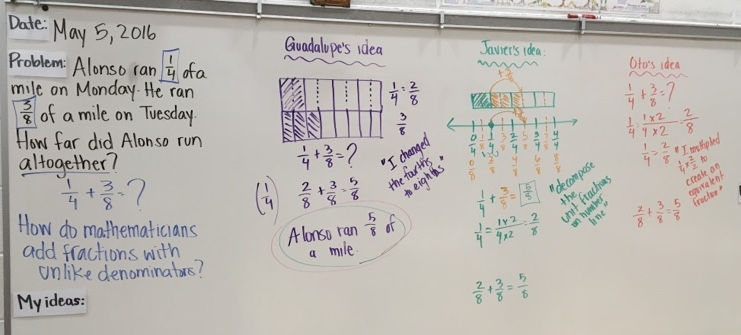Select PageA surprisingly difficult but critically important part of designing a lesson is establishing the learning goals. Many teachers write learning goals in the form of behavioral outcomes: “Students will be able to…” Frequently this is what their administrators want, and sometimes it is what they were taught to do in their preservice training.

But learning in an academic context is a mental activity, and so learning goals for academic subjects should actually describe a desired internal change in the student. That is, at the end of a lesson, students should understand something new, should know something knew, or should feel differently about something. Their ability to DO something new is a side effect of their new knowledge or understanding.

Articulating learning goals in this way helps you think more deeply about the contents you are trying to teach. To see how this is true, let’s try rephrasing a typical learning goal for 5th grade: “Students will be able to add fractions with unlike denominators.” Contrast the following possible learning goals, expressed as internal changes in the student, either of which would yield the same ability to add fractions correctly:

(A) The student knows that to add two fractions with different denominators, one needs to find the least common multiple of the two denominators, convert each fraction to an equivalent fraction with that least common multiple (called a ‘common denominator’), then add the numerators of the converted fractions, and write the result as (sum of numerators) / (common denominator).

vs.

(B) The student understands that, because the denominator of a fraction defines the size of the unit, fractions expressed using different units (e.g. fourths and thirds) must be converted to a common unit (e.g. twelfths) before they can be added. The student will realize that she can use her previous knowledge of equivalent fractions to rewrite the fractions with a common unit, and then add those fractions in the normal way. Students will realize that the idea of least common multiple can be used to find the smallest common unit.

Goal A is all about a new procedure for a new type of problem, addition of fractions with unlike denominators. The student knows what to do, but not necessarily why, except that you have to have a common denominator (for some reason). Goal B, on the other hand, is about connecting a new type of problem to knowledge the student already has about the meaning of the denominator, about equivalent fractions, and about least common multiples. Lessons designed around these different goals will necessarily be very different.

Consider also how a student would feel after a lesson designed around one or the other of these goals. For goal A, the student might feel burdened with another procedure she has to remember and be careful to use in the right circumstances. For goal B, the student might feel a sense of power – she can use what she already knows to solve a new problem!

Then consider how you would assess students vis-à-vis these goals. A worksheet or quiz that asks students to add fractions with unlike denominators might tell you whether students know what to do, but won’t tell you if they understand why.

The point of this is not that goal B is a better goal – although clearly I think it is – but to illustrate how important it is to think beyond observable behaviors and identify the internal mental changes you wish to make in the student as you design a lesson, and as you plan for assessment.